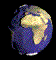How is a fourth dimension visible?

"Feynman was continually bothered by the way that physical behavior could be expressed in algebraic conventions without concrete visualization: 'Strange! I don't understand how it is that we can write mathematical expressions and calculate what the thing is going to do without being abe to picture it.'

Richard Feynman"The same dilemma faced anyone, artist or scientist, when, when they aspired to depict more than three dimensions–namely our lack of representational resources to depict four- or n -dimensional space."

Kemp, visualizations, p100.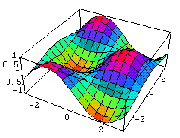Depiction of a four dimensional cube.
Super strings are hypothetical substratum.
Are strings the underlying lattice?

"Feynman diagrams look superficially like the simple graphics that physicists have used for centuries, but they are devices of exceptional power.

Feynman's diagrams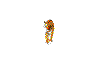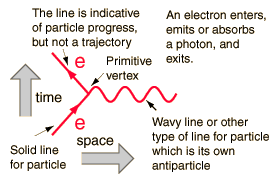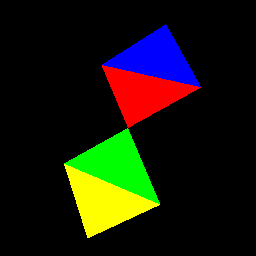"The diagrams mirror his conviction that

'there is . . . a rhythm and a pattern between the phenomena of nature which is not apparent to the eye, but only to the eye of analysis'."

Kemp on Feynman, Visualizations, p. 101.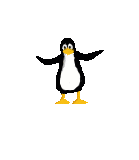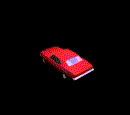"In the 1949 paper which inaugurated his [Feynman's] method, he provided the the classic demonstration of the 'fundamental interactions' in which electrons exchange a photon. The electrons are figured as solid lines, while the process of exchange of a 'virtual quantum' is represented by a wavy line, which graphically signals that the photon can be emitted from one electron and absorbed by the other , or, if it travels backward in time, in reverse. Either way, the result is the deflection of both electrons, denoted by the angular deviation."

p. 101.

Descibing the unseen aspects of the world.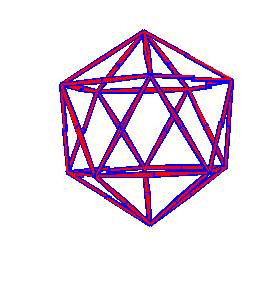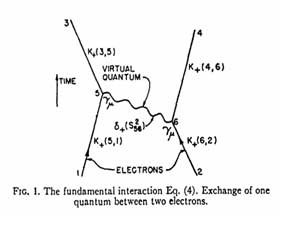Shown to the left, these so-called Feynman diagrams were introduced by Richard Feynman in the journal Physical Review in 1949, and they quickly became an essential tool for particle physicists.

The first published example of what is now called a Feynman diagram appeared in Feynman's 1949 Physical Review article [see image labeled fig 1]. It depicted the simplest contribution to an electron-electron interaction, with a single virtual photon (wavy line) emitted by one electron and then absorbed by the other. In Feynman's imagination--and in the equations--this diagram also represented interactions in which the photon is emitted by one electron and travels back in time to be absorbed by the other, which is allowed within the Heisenberg time uncertainty.

see Feynman diagrams: The Science of Doodling.